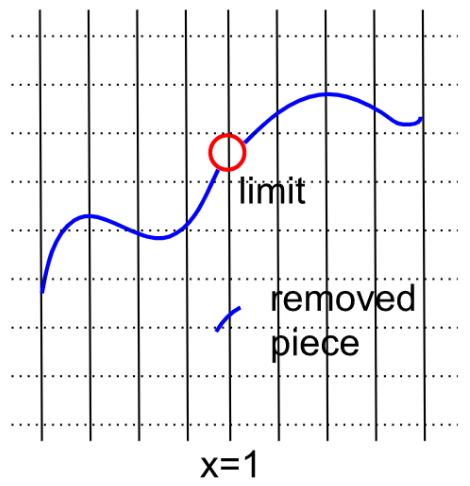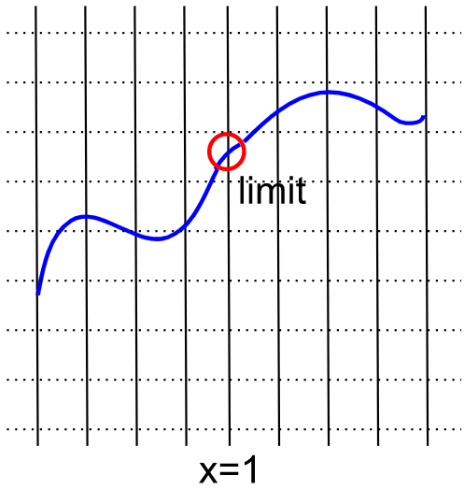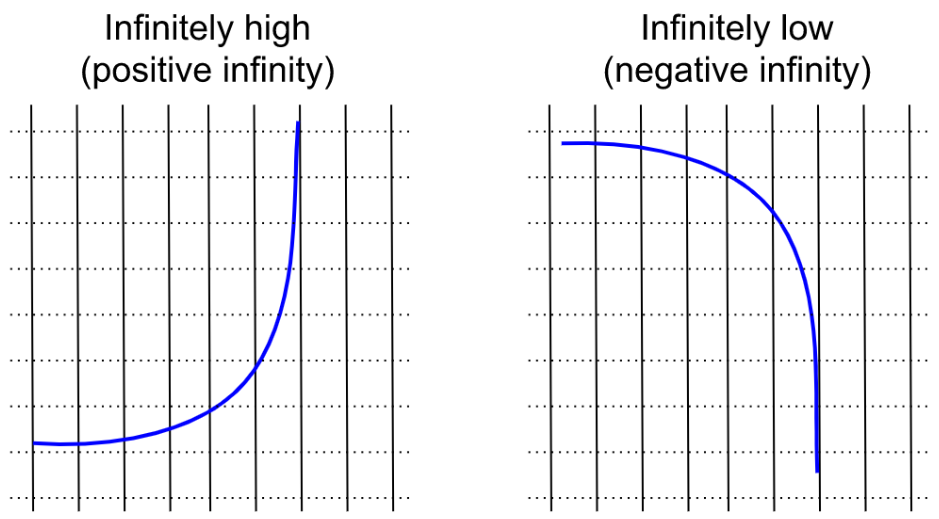# Intuiting Limits

This post is part of a series.

The limit of a function is the height, or $y$-value, where it looks like the scribble is going to hit a particular vertical line, or $x$-value – but sometimes scribbles don’t actually hit where they look like they’re going to hit.

For example, if you have a weird scribble with a broken piece removed, the limit at $x=1$ is the height where the rest of the function suggested the piece was going to hit, not the “island” segment where the piece actually hits $x=1.$On the other hand, the function below actually occupies its limit at $x=1,$ because it hits where it looks like it’s going to hit.Functions that actually hit where they look like they’re going to hit, like the one above, are called continuous functions. A rule of thumb: a function is continuous if you can draw it without picking up your pen.

A faster way to find a function’s limit, without having to draw out the function, is to use the function’s equation. If the function is continuous, then all you need to do is plug the $x$-value of the limit into the function. For example, to find the limit of $x^2-1$ at $x=1,$ you can simply plug in $1$ for $x{:}$

\begin{align*} \lim_{x\rightarrow 1}(x^2-1)=1^2-1=0 \end{align*}

But sometimes you’re not able to plug in directly, and you have to manipulate the equation first. For example, if you try plugging in to $\lim\limits_{x \rightarrow 1} \frac{x^2-1}{x-1},$ you get $\frac{0}{0},$ which is undefined because of the 0 in the denominator. But if you manipulate the equation first, you can convert it into a form where you can plug in the limit:

\begin{align*} \lim_{x \rightarrow 1} \frac{x^2-1}{x-1} &= \lim_{x \rightarrow 1} \frac{(x+1)(x-1)}{x-1} \\[5pt] &= \lim_{x \rightarrow 1} x+1 \\[5pt] &= 1+1 \\[5pt] &= 2 \end{align*}

Other times, the limit might not even exist! In $\lim\limits_{x \rightarrow 1} \frac{1}{\vert x-1 \vert},$ you cannot plug in because you’ll get $\frac{1}{0},$ which is undefined. However, there is no way to manipulate the function as it is already in its most simplified form. When a limit doesn’t exist, it means there is no number the function looks like it should go to at that $x$-value.

Sometimes this just happens because a function gets infinitely high or infinitely low. To discern this from the equation, it is helpful to think of plugging in $x$-values that are very close, but not equal to, the limit value.

For example, in $\lim\limits_{x \rightarrow 1} \frac{1}{\vert x-1 \vert},$ you might try plugging in $0.999$ instead of $1.$ Then you get

\begin{align*} \frac{1}{\vert 1-0.999 \vert} = \frac{1}{0.001} = 1000. \end{align*}

If you try an $x$-value that is even closer, like $0.999999,$ then you get

\begin{align*} \frac{1}{\vert 1-0.999999 \vert} = \frac{1}{0.000001} = 1000000, \end{align*}

which is an even bigger number. The function just keeps getting higher, the closer the $x$-value gets to $1.$

The same thing happens if we approximate from the other side with numbers slightly larger than $1,$ such as $1.001$ and $1.000001.$ So even though the limit doesn’t exist, we say that the limit is infinity. That’s written as $\lim\limits_{x \rightarrow 1} \frac{1}{\vert 1-x \vert} = \infty.$Other times, though, the limit will look like something when we approximate with numbers slightly smaller than $x,$ and something totally different when we approximate with numbers slightly bigger than $x.$ In these cases, the limit just doesn’t exist, and there’s nothing we can do about it.

For an example, consider $\lim\limits_{x \rightarrow 1} \frac{1}{1-x},$ which is like the previous example but without the absolute value signs. If we try to approximate the limit using numbers slightly smaller than $1,$ the function just keeps getting higher and higher, to positive infinity.

\begin{align*} x=0.999 &\Longrightarrow \frac{1}{1-x}=1000 \\[5pt] x=0.999999 &\Longrightarrow \frac{1}{1-x}=1000000 \end{align*}

But if we use numbers slightly bigger than $1,$ the function just keeps getting lower and lower, to negative infinity.

\begin{align*} x=1.001 &\Longrightarrow \frac{1}{1-x}=-1000 \\[5pt] x=1.000001 &\Longrightarrow \frac{1}{1-x}=-1000000 \end{align*}

Since we get two different answers depending on whether we approach $1$ from above or below, the limit does not exist.

This post is part of a series.

Tags: